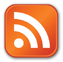Counting days between two dates - programming in Java or C

When I was a Computer Science student at Kasetsart University, I had this ANSI C programming homework to make a program that counts the number of days between dates, at that time I didn't have a computer yet, I had to write this code on a piece of papaer while at the hostel - then write it again on the computer at university or at my cousin's who had one. Now, many years later, that same code became part of the j2me DaysToDay Calendar Counter Program - I tried using the java Calendar class on mobile but it had bugs/wrong_results while computing long date differences of many many years. Here's the main part of the code, the full Code is here.

long dtd(int d1, int m1, int y1, int d2,int m2,int y2)
{
long  totald, dofyrsbtw, stm2;
int  n, btw;
if( ( y2 < y1) || ( y1 == y2 && m2 < m1 ) || ( y1 == y2 && m1 == m2 && d2 < d1) )
{

int trans;
trans = y1;
y1 = y2;
y2 = trans;
trans = m1;
m1 = m2;
m2 = trans;
trans = d1;
d1 = d2;
d2 = trans;
}
if ( y1 == y2)
{
if( m1 == m2 )
totald = d2 - d1;
else
{
int t1m1 = m1;
btw = 0;
while(true)
{
if( ( m2 - t1m1) == 1)
break;
btw += mlength(++t1m1, y1);
}
totald = mlength(m1, y1) - d1 + d2 +btw;
}

}
else
{
int t2m1, m1tend, t1y1;
t2m1 = m1;
m1tend = 0;
while(true)
{
if(t2m1 == 12)
break;
m1tend += mlength(++t2m1, y1);
}
dofyrsbtw = 0;
t1y1 = y1;
while(true)
{
if( y2 - t1y1 == 1)
break;
dofyrsbtw += ylength( ++t1y1);
}
stm2 = 0;
n = 0;
while(true)
{
if(m2 == n + 1)
break;
stm2 += mlength(++n, y2);
if(n == m2 - 1 )
break;
}
totald = mlength(m1, y1) - d1 + m1tend + dofyrsbtw + stm2 + d2;
}

return(totald);

}

int mlength(int m, int y)
{
int retml = 0;
switch(m)
{
case 1 :  retml = 31;break;
case 2 :  if(  (y%4 == 0  &&  y%100 != 0) || ( y%400 == 0)  ) retml = 29 ; else retml = 28;break;
case 3 :  retml = 31;break;
case 4 :  retml = 30;break;
case 5 :  retml = 31;break;
case 6 :  retml = 30;break;
case 7 :  retml = 31;break;
case 8 :  retml = 31;break;
case 9 :   retml = 30;break;
case 10 :  retml = 31;break;
case 11 :  retml = 30;break;
case 12 :  retml = 31;break;
}
return(retml);
}
boolean check(int d, int m, int y)
{
return ( ( m>0 && m < 13) && ( (d>0) && (d <= mlength(m, y))) );
}
int ylength( int y)
{
int doy;
if(  (y%4 == 0  &&  y%100 != 0) || ( y%400 == 0)  ) doy = 366; else doy = 365;
return(doy);
}